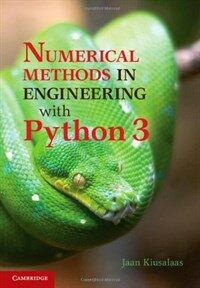> 상세정보

# 상세정보## Numerical methods in engineering with Python 3 (8회 대출)

자료유형
단행본
개인저자
Kiusalaas, Jaan.
서명 / 저자사항
Numerical methods in engineering with Python 3 / Jaan Kiusalaas.
발행사항
Cambridge ;   New York :   Cambridge University Press,   c2013.
형태사항
xi, 423 p. : ill. ; 26 cm.
ISBN
9781107033856
요약
"This book is an introduction to numerical methods for students in engineering. It covers solution of equations, interpolation and data fitting, solution of differential equations, eigenvalue problems and optimisation. The algorithms are implemented in Python 3, a high-level programming language that rivals MATLAB in readability and ease of use. All methods include programs showing how the computer code is utilised in the solution of problems. The book is based on Numerical Methods in Engineering with Python, which used Python 2. This new edition demonstrates the use of Python 3 and includes an introduction to the Python plotting package Matplotlib. This comprehensive book is enhanced by the addition of numerous examples and problems throughout"--
일반주기
Includes index.
내용주기
Machine generated contents note: 1. Introduction to Python; 2. Systems of linear algebraic equations; 3. Interpolation and curve fitting; 4. Roots of equations; 5. Numerical differentiation; 6. Numerical integration; 7. Initial value problems; 8. Two-point boundary value problems; 9. Symmetric matrix eigenvalue problems; 10. Introduction to optimization.
일반주제명
Engineering mathematics --Data processing. Python (Computer program language).
 000 00000cam u2200205 a 4500 001 000045899849 005 20170317141736 008 170317s2013 enka 001 0 eng d 010 ▼a 2012036775 020 ▼a 9781107033856 035 ▼a (KERIS)REF000016987855 040 ▼a DLC ▼b eng ▼c DLC ▼e rda ▼d DLC ▼d 211009 050 0 0 ▼a TA345 ▼b .K58 2013 082 0 0 ▼a 620.00285/5133 ▼2 23 084 ▼a 620.00285 ▼2 DDCK 090 ▼a 620.00285 ▼b K62n 100 1 ▼a Kiusalaas, Jaan. 245 1 0 ▼a Numerical methods in engineering with Python 3 / ▼c Jaan Kiusalaas. 260 ▼a Cambridge ; ▼a New York : ▼b Cambridge University Press, ▼c c2013. 300 ▼a xi, 423 p. : ▼b ill. ; ▼c 26 cm. 500 ▼a Includes index. 505 8 ▼a Machine generated contents note: 1. Introduction to Python; 2. Systems of linear algebraic equations; 3. Interpolation and curve fitting; 4. Roots of equations; 5. Numerical differentiation; 6. Numerical integration; 7. Initial value problems; 8. Two-point boundary value problems; 9. Symmetric matrix eigenvalue problems; 10. Introduction to optimization. 520 ▼a "This book is an introduction to numerical methods for students in engineering. It covers solution of equations, interpolation and data fitting, solution of differential equations, eigenvalue problems and optimisation. The algorithms are implemented in Python 3, a high-level programming language that rivals MATLAB in readability and ease of use. All methods include programs showing how the computer code is utilised in the solution of problems. The book is based on Numerical Methods in Engineering with Python, which used Python 2. This new edition demonstrates the use of Python 3 and includes an introduction to the Python plotting package Matplotlib. This comprehensive book is enhanced by the addition of numerous examples and problems throughout"-- ▼c Provided by publisher. 650 0 ▼a Engineering mathematics ▼x Data processing. 650 0 ▼a Python (Computer program language). 945 ▼a KLPA

### 소장정보

No. 소장처 청구기호 등록번호 도서상태 반납예정일 예약 서비스
No. 1 소장처 청구기호 620.00285 K62n 등록번호 121239620 도서상태 대출가능 반납예정일 예약 서비스

### 컨텐츠정보

#### 목차

`1. Introduction to Python; 2. Systems of linear algebraic equations; 3. Interpolation and curve fitting; 4. Roots of equations; 5. Numerical differentiation; 6. Numerical integration; 7. Initial value problems; 8. Two-point boundary value problems; 9. Symmetric matrix eigenvalue problems; 10. Introduction to optimization.`

### 관련분야 신착자료

#### 공학수학 문제풀이

Zill, Dennis G. (2022)

#### Design, fabrication and characterization of multifunctional nanomaterials

Thomas, Sabu (2021)

#### 통계적 품질관리

Montgomery, Douglas C. (2022)

#### 재료과학과 공학

Callister, William D. (2021)

김도영 (2022)

#### Multiscale modeling approaches for composites

Chatzigeorgiou, George (2022)

장경욱 (2022)

#### Current research in neuroadaptive technology

Fairclough, Stephen H (2022)

#### 재료공학개론

Shackelford, James F. (2022)

이지웅 (2021)

이정원 (2022)

유봉조 (2022)

송철기 (2022)

이시중 (2022)

#### 공학수학

Zill, Dennis G. (2022)

#### (Beer의) 재료역학

Beer, Ferdinand Pierre (2022)